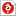검색어 입력폼

# [알고리즘]자료구조] n-queen problem

등록일 2006.03.26한컴오피스 (hwp) | 3페이지 | 가격 500원

## 소개글

Implement the n-queens problem

C++

## 본문내용

Implement the n-queens problem
Do the followings: For n = 4, 5, 6, 7, 8, 9, 10, 11, 12, 13, 14,15
1. Output the positions of queens for the n-queens problem.
2. Count the number of promising nodes before we find a solution of nqueen
problem.
3. Check the running time of each case of n-queens problem, draw a graph
with the running time, and do analysis the time complexity of the
algorithm.

없음